# 雅克比迭代算法(Jacobi Iterative Methods) -- [ mpi , c++]

$$a_{11}*x_{1} + a_{12}*x_{2} + a_{13}*x_{3} = b_{1}$$
$$a_{21}*x_{1} + a_{22}*x_{2} + a_{23}*x_{3} = b_{2}$$
$$a_{31}*x_{1} + a_{32}*x_{2} + a_{33}*x_{3} = b_{3}$$

$$x_{1}=\frac{１}{a_{１1}}(b_{１} -a_{１2}*x_{２} -a_{１3}*x_{3})$$
$$x_{２}=\frac{１}{a_{21}}(b_{2} -a_{2１}*x_{１} -a_{23}*x_{3})$$
$$x_{３}=\frac{１}{a_{３1}}(b_{３} -a_{３１}*x_{１}-a_{３２}*x_{２})$$

$$x^{k}=(X_{1}^{k},X_{2}^{k},X_{3}^{k})$$ , $$k = 1,2,3...n$$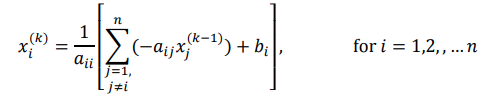eg: 对于方程组：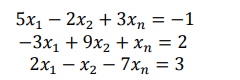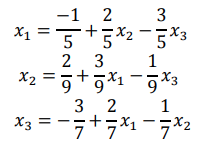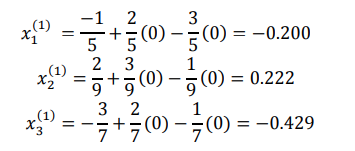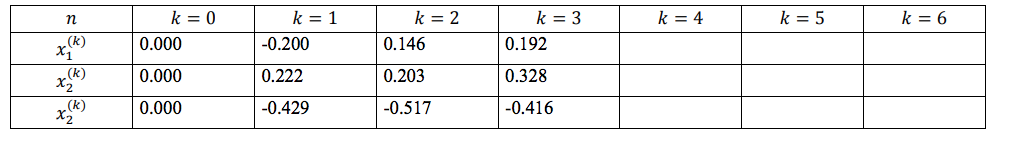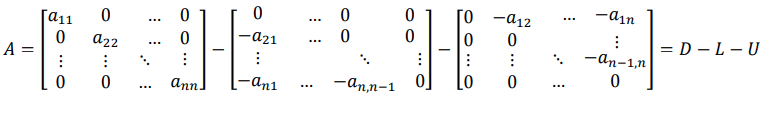x = b ,然后我们就可以得到：
Dx = b + (L+U)x ，当我们得到这个公式的时候，求解D的逆矩阵就容易了很多，我们得到D的逆矩阵为：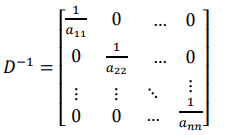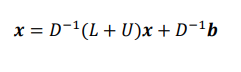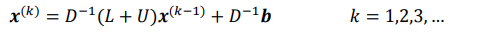$$T = D^{-1}*( L +U)$$ , $$c = D^{-1}*b$$ ;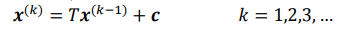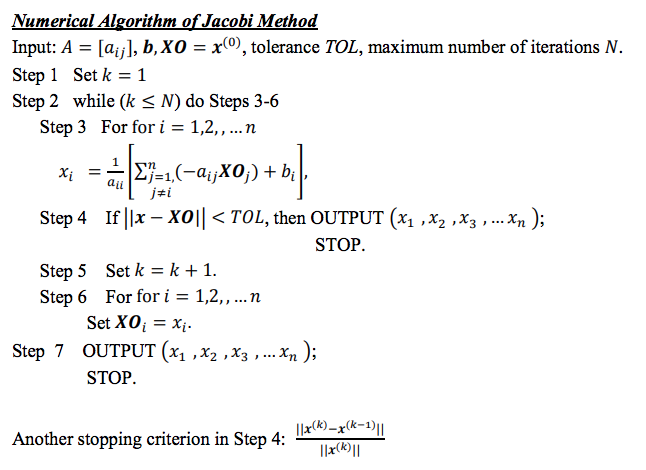posted @ 2018-12-20 14:52  龚细军  阅读(4394)  评论(0编辑  收藏  举报